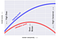While developing machine learning models, machine learning practitioners usually encounter the problem of selecting the best model.

Generally, the best model is the one with the least possible prediction error. So in order to find the best model we need to minimize the prediction error. The prediction error for any machine learning algorithm can be broken down into

• Irreducible Error
• Bias
• Variance

## Irreducible Error

Irreducible errors are those errors that cannot be reduced irrespective of any algorithm that you use in that model. It is caused by unusual variables that have a direct influence on the output variable. This error cannot be reduced or minimized.

In order to make the model “the best” we are left with reducible errors i.e Bias and Variance.

## Bias

Bias is basically how far we have predicted the value from the actual value. It can also be defined as the difference between the predicted value and the expected value.

High bias will cause the model to miss a relationship between the input and output variable. Also, it is assumed that the model is quite simple and doesn’t feed the complexity of the data to determine the relationship and thus causing underfitting.

For high-bias models, the performance of the model on the validation set is similar to the performance on the training set.

## Variance

Variance is used to explain exactly how scattered the predicted values are from the actual values. A high variance in a dataset means that the model has trained with a lot of noise and irrelevant data thus causing the overfitting in the model.

For high-variance models, the performance of the model on the validation set is far worse than the performance on the training set.

“Increasing the bias will decrease the variance. And increasing the variance will decrease the bias”

## How exactly bias and variance affect machine learning models?

We can put the relationship between bias and variance in four categories

1. High Variance and High Bias: Models will be inconsistent and also inaccurate on average.
2. High Variance and Low Bias: Models will be somewhat accurate but inconsistent on average.
3. Low Variance and High Bias: Models will be consistent but will perform very low on average.
4. Low Variance and Low Bias: It is the ideal scenario, models will be consistent and accurate on average.

If the model is too simple and has very few parameters it will suffer from high bias and low variance and on another hand, if the model has a large number of parameters it will have high variance and low bias.

Fundamentally, the question of “the best model” is about finding a sweet spot in the trade-off (an act of balancing between two opposing situations) between bias and variance.

As in machine learning, the ideal algorithm has low bias and can accurately model the true relationship and it has low variability, by producing consistent predictions across different datasets.

Finding a trade-off is basically a way to make sure that the model is neither overfitted nor under fitted in any case.A schematic of the relationship between model complexity, training score,and validation score

The conclusion from the above plot

• The training score is everywhere higher than the validation score. This is generally the case: the model will be a better fit to data it has seen than to data it has not seen.
• For very low model complexity (a high-bias model), the training data is under-fitted, which means that the model is a poor predictor both for the training data and for any previously unseen data.
• For very high model complexity (a high-variance model), the training data is overfitting, which means that the model predicts the training data very well, but fails for any previously unseen data.
• For some intermediate values, the validation curve has a maximum value. This level of complexity indicates a suitable trade-off between bias and variance.

## Methods to find the tradeoff

• Regularization
• Dimensionality Reduction
• Ensemble learning like Bagging or Boosting

## Summary

Bias and Variance play an important role in determining which forecast model to use. A good model would be one where both the bias and the variance are low.

In reality, we cannot calculate the real bias and variance because we do not know the actual underlying target function (a function that can be used to predict results). Nevertheless, bias and variance provide the tools to understand the behavior of machine learning algorithms in the pursuit of predictive performance.

However, we should always aim for a model where the model’s score for training and validation data is as close as possible.